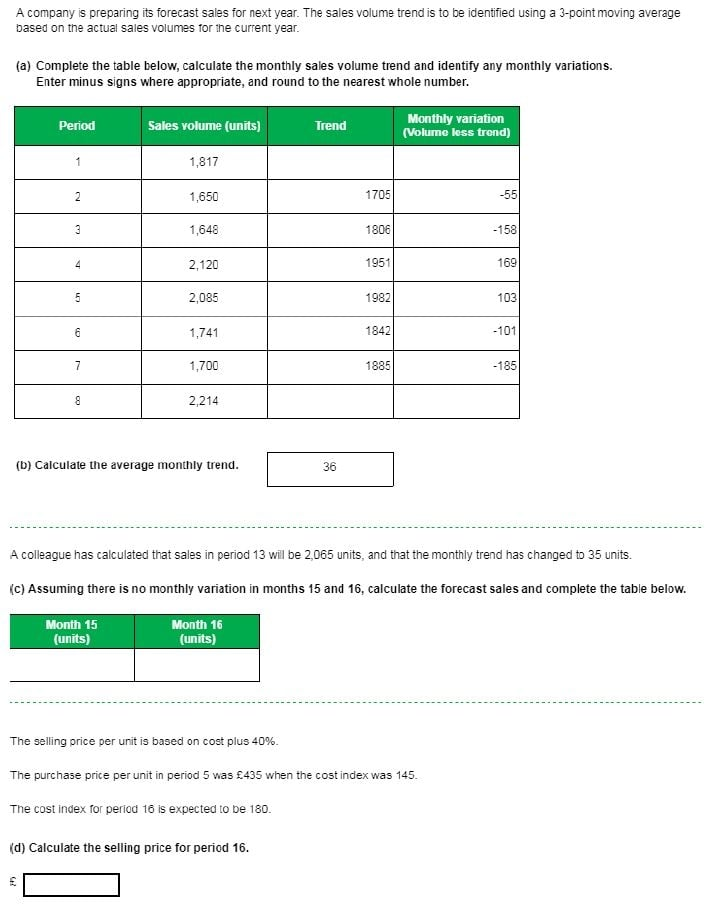# Cash Management CBT 2 Task 2 - Sales forecasting and trend

Hi All,

There is one question out of the two Cash Management (2013) assesments that I cannot for the life of me get to the bottom of.
It is task 2 part c) & d) calculating forecasted sales. All of the scripts I have read have a pattern of variation and can be worked out easily whereas with this question we don't.

If anybody could help I would be SO SO grateful. I can do part a) & b) no problem but c) and d) not so promising.• (c) So for period 13 = 2065 units

The monthly trend is 35, so all you do is keep adding 35 for the next period, so would be the following:

Period 14 - 2065 + 35= 2100 (take the 2065 from what is given in the question)
Period 15 - 2100 +35 = 2135 units
Period 16 - 2135 + 35 = 2170 units

(d) This covers the mark up/margin section and indexing - I would suggest you learn this as these are easy marks to get in the exam.

From the question, you can tell this is a mark-up, as the selling price is based on cost PLUS 40%, which is equivalent to 140%.

As the index equation goes, it is current year/base year for indexing -
(1)180/145 = 1.241737931
(2) 1.24 x 435 = £540

As this only represents only 100%, we need to add the 40%, so you simply multiply by 140 =
(3) 540 x 1.4 = £756

Hope this makes sense
• Aww, Sabina!

Thank you so so so so much for coming back to me, that was very kind of you. Since writing the post I managed to work out part c) but I really appreciate it and your explanation of part d) was so helpful, I understand markup and margin but agree that I need to remind myself how to work out indexing, it all makes perfect sense and I will remeber this for my exam.

Thanks again!